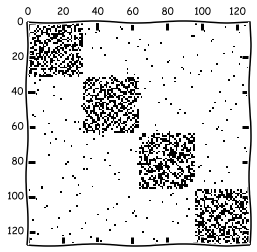# Community detection algorithms¶

On this seminar your are asked to implement simple community detection algorightm. It is called Markov Cluster Algorithm (MCL).

It consists of the following steps: <br> Input: Transition matrix $T = D^{-1}A$ <br> Output: Adjacency matrix $M^*$ <br> <br>

1. Set $M = T$
2. repeat:
1. Expansion Step: $M = M^p$ (usually $p=2$)
2. Inflation Step: Raise every entry of $M$ to the power $\alpha$ (usualy $\alpha=2$)
3. Renormalize: Normalize each row by its sum
4. Prunning: Remove entries that are close to $0$
3. until $M$ converges
4. $M^* = M$ <br> <br>

As a result you should get a cluster matrix s.t. elements of the cluster correspont to nonzero elements of the rows of the matrix. <br>

• Run this method for network 1, 2 and 3.
In :
import numpy as np
import pandas as pd
import matplotlib.pyplot as plt
plt.xkcd()
import networkx as nx
import scipy
import scipy.io
%matplotlib inline

In :
data = scipy.io.loadmat('network1.mat')
A = data['A'].astype('float')
plt.spy(A)
comm = data['Comm']
# Continue here
#In :
def MCL(A, tol, p, alpha):
step = 1
col_sums = A.sum(axis = 0)
T = A / col_sums[np.newaxis, :]
M = T
while(1):
print 'step ', step
step += 1
# Expancion step:
M1 = np.linalg.matrix_power(M, p)
# Inflation step:
M1 = np.power(M1, alpha)
col_sums = M1.sum(axis = 0)
M1 = M1 / col_sums[np.newaxis, :]
M1[M1<=tol] = 0
if np.linalg.norm(M - M1) == 0:
return M1
else:
M = M1.copy()